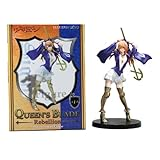Sigui Figure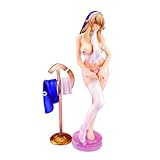Sigui Figure (Excellent Model)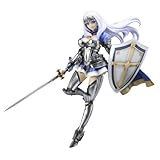Annelotte Figure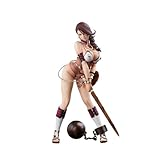Branwen Figure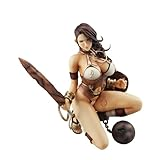Branwen Figure (Excellent Model)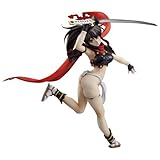Izumi Figure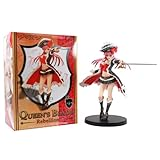Liliana Figure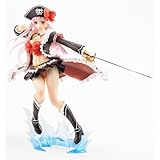Liliana Figure [Good Smile]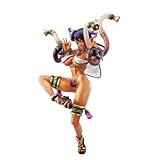Luna Luna FigureMelona Figure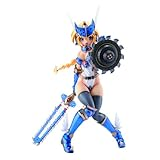Mirim FigureRisty Figure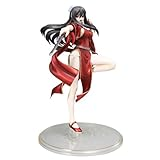Tarnyang Figure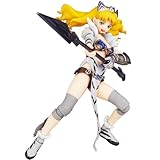Elina Figure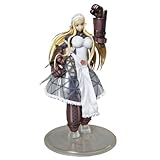Vante Figure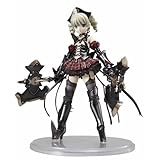Ymir Figure